(248)601-6400 support@rentviewer.com

Had a meeting yesterday with a company that wants to order a custom dashboard. I asked how they currently get their metrics, and they showed me screenshots of their scorecard.

Their process involves exporting numbers from the property management system into Excel and then calculating averages by Regional Manager and Property Manager.

I pointed out a flaw in the way they were calculating the Average Rent per Square Foot and the Average Vacancy. Details below.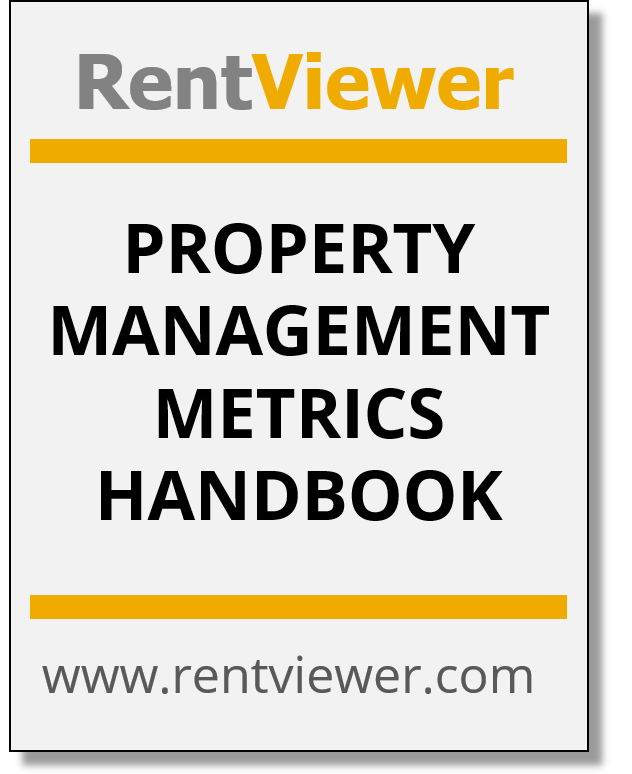## Average Rent Per Square Foot

One metric they track is Average Rent Per Square Foot of an entire building. Any property management system can give you that number.

However, since some units in each building are not rentable, they have to exclude these units and arrive at the calculation manually.

Their Average Rent Per Square Foot calculation looks like this: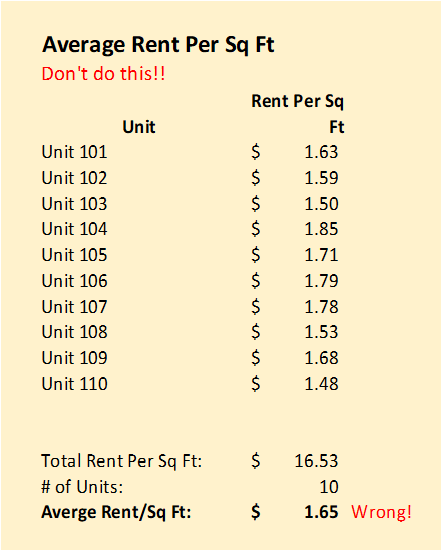## Metrics that are ratios cannot be added up!

The above calculation for the average Rent per Square Foot, \$1.65, is wrong.

## Rent per Square Foot Calculation

The right way for calculating metrics that are ratios, such as Rent per Square Foot,  is to start with their components:
For Rent Per Sq Foot, the components are Total Rent and Total Square Feet for each unit.

To get the correct answer, you have to know the square footage of each unit and the rent of each unit. The correct Rent per Square Foot is calculated by dividing the total rent by the total square footage. See further below below for more detail.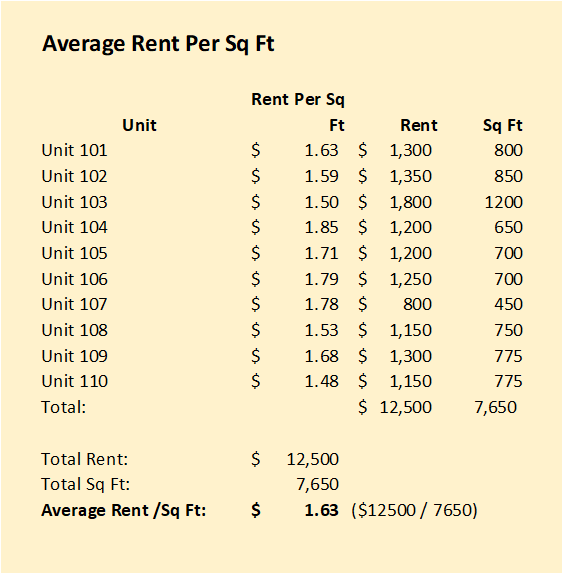## Average Vacancy Rate

Vacancy of a building is simply the number of vacant units divided by the total rentable units. In this case, the property management system is providing the vacancy % of each building. Then, these are being averaged for selected buildings as shown below: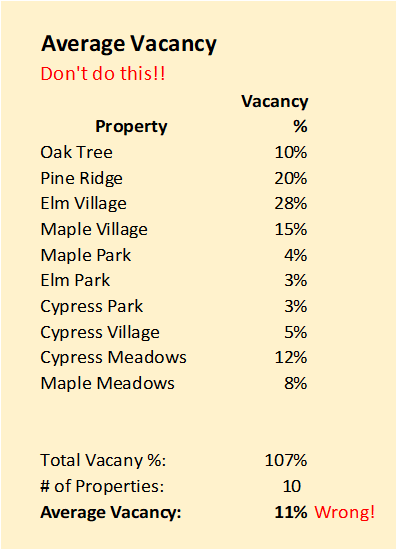The correct answer way to calculate the average vacancy of a group of properties to divide the total number of vacant units (for all building) and total number of rentable units.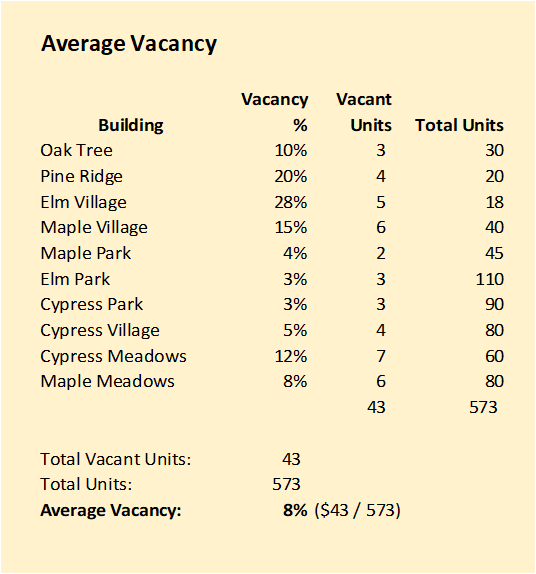## Don’t Add Averages and Ratios Across Properties

The right way for calculating such metrics is to start with their components:
For Rent Per Sq Ft, the components are Total Rent and Total Square Feet for each unit

For the Average Vacancy, the components are Total Vacant Units and Total Rentable Units.

Other examples where such errors can be made are …
Average Cost Per Lead: Suppose you have been provided the average cost per lead from Zillow, Apartments.com and Craigslist, and you want to know the average across all three sources. Don’t simply take the average of the three numbers. You need to know how many leads you got from each one, and how much you spent on each site.